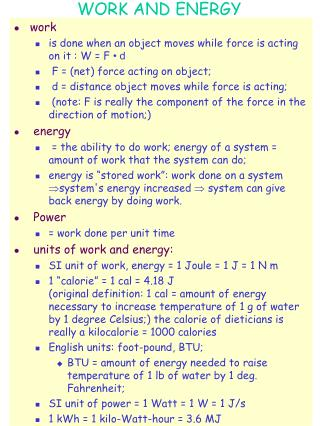DownloadDownload PresentationWORK AND ENERGY

# WORK AND ENERGY

Download Presentation## WORK AND ENERGY

- - - - - - - - - - - - - - - - - - - - - - - - - - - E N D - - - - - - - - - - - - - - - - - - - - - - - - - - -
##### Presentation Transcript

1. WORK AND ENERGY • work • is done when an object moves while force is acting on it : W = F • d • F = (net) force acting on object; • d = distance object moves while force is acting; • (note: F is really the component of the force in the direction of motion;) • energy • = the ability to do work; energy of a system = amount of work that the system can do; • energy is “stored work”: work done on a system system's energy increased  system can give back energy by doing work. • Power • = work done per unit time • units of work and energy: • SI unit of work, energy = 1 Joule = 1 J = 1 N m • 1 “calorie” = 1 cal = 4.18 J (original definition: 1 cal = amount of energy necessary to increase temperature of 1 g of water by 1 degree Celsius;) the calorie of dieticians is really a kilocalorie = 1000 calories • English units: foot-pound, BTU; • BTU = amount of energy needed to raise temperature of 1 lb of water by 1 deg. Fahrenheit; • SI unit of power = 1 Watt = 1 W = 1 J/s • 1 kWh = 1 kilo-Watt-hour = 3.6 MJ

2. POTENTIAL AND KINETIC ENERGY • lifting object: • work done against gravitational force;raised object can drop down and do work (e.g. pull a cart) • i.e. raising object (doing work on it), increased its potential to do work  “gravitational potential energy”; • falling of raised object: • object is accelerated -- loses potential energy -- gains energy of motion - “kinetic energy”; • object can do work by virtue of its motion. • quantitatively: • W = F h, F = m g  W = m g h • let object drop: kinetic energy K = mv2 /2 • conservation of (mechanical) energy: • when lifting the object, its gravitational potential energy is increased by the amount of work done lifting; • when the object falls, this energy is converted (transformed) into “kinetic energy” (energy of motion) • gravitational potential energy: Ug = m g h • kinetic energy K = mv2 /2

3. TYPES OF ENERGY • Many different kinds of energy; can be transformed back and forth into each other: • kinetic energy = energy of motion = work that system can do because of its motion; (translational or rotational) • potential energy = energy of position or state; (gravitational, elastic, electric, chemical, nuclear) • gravitational energy = work system can do due to objects having been raised against gravitational force; depends on “reference level” i.e. on how far object can fall down; • elastic energy due to ability of deformed (stretched, squeezed,..) system to snatch back (e.g. rubber band, spring..) • thermal energy = kinetic energy of random motion of molecules; brought into system by “heating”; different from other forms of energy - not all of it can be converted back. • electromagnetic energy (electric energy) = energy due to electromagnetic forces; • radiant energy = energy carried by electromagnetic radiation; • chemical energy = energy stored in molecular structure of chemical compounds; can be “liberated” by chemical reactions converting compound into other compounds with less stored chemical energy. • nuclear energy = energy due to nuclear structure, i.e. how protons and neutrons are bound to each other to form nuclei.

4. CONSERVATION OF ENERGY • Energy conservation: • the total energy of all participants in any process is unchanged throughout that process. Energy can be transformed (changed from one energy form to another), and transferred (moved from one place to another), but cannot be created or destroyed. In an isolated system the total amount of energy is conserved. • Conservation laws in physics: • “conserved quantities”: = quantities that do not change - “are conserved” • Conservation laws are related to “symmetry” property of system -also called “invariance” property. • Every invariance property is associated with a conserved quantity. • Energy conservation is related to “invariance under translation in time” (i.e. laws of physics do not change as time passes). • Other conserved quantities: • momentum (invariance under translation in space); • angular momentum (rotation); • electric charge (“gauge transformation”); • certain properties of subatomic particles (e.g. “Isospin”, “color charge”, ...)